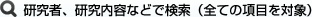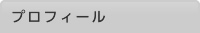#研究者総覧## 谷内　靖　　タニウチ ヤスシ

教員組織 電話番号 学術研究院（理学系）理学部　数学科　数理科学コース 教授## プロフィール

1998 , 名古屋大学 , 多元数理科学研究科
1995 , 名古屋大学 , 工学研究科 応用物理学専攻

1993 , 名古屋大学 , 理学部 物理学科

2012- , 信州大学　理学部　教授
2005- , 信州大学　理学部　助教授
2000- , 日本学術振興会 特別研究員
2000- , 信州大学　理学部 助手
1998- , 名古屋大学大学院　多元数理科学研究科 研究生

## 研究活動業績

A remark on the uniqueness of Kozono-Nakao's mild $L^3$-solutions on the whole time axis to the Navier-Stokes equations in unbounded domains
Partial Differ. Equ. Appl.,(68) 2021
Author:Taniuchi, Yasushi

Brezis-Gallouet-Wainger type inequality and its application to the Navier-Stokes equations
Contemp. Math.,710:211-222 2018
Author:NAKAO, K; TANIUCHI, Y

Brezis-Gallouet-Wainger type inequalities and blow-up criteria for Navier-Stokes equations in unbounded domains
COMMUNICATIONS IN MATHEMATICAL PHYSICS,3(359):951-973 2018
Author:NAKAO, K; TANIUCHI, Y

An alternative proof of logarithmically improved Beale-Kato-Majda type extension criteria for smooth solutions to the Navier-Stokes equations
Nonlinear Analysis,176:48-55 2018
Author:Nakao, K and Taniuchi, Y

Existence of solutions on the whole time axis to the Navier-Stokes equations with precompact range in L^3
Archiv der Mathematik,104(6):539–550 2015
Author:Reinhard FARWIG, Tomoyuki NAKATSUKA, Yasushi TANIUCHI

Uniqueness of solutions on the whole time axis to the Navier-Stokes equations in unbounded domains
Comm.Partial Differential Equations,40(10):1884-1904 2015
Author:Reinhard FARWIG, Tomoyuki NAKATSUKA, Yasushi TANIUCHI

Uniqueness of backward asymptotically almost periodic-in-time solutions to Navier-Stokes equations inunbounded domains
Discrete and Continuous Dynamical System Ser.S,6(5):1215-1224 2013
Author:Farwig, R; Taniuchi, Y

Uniqueness of almost periodic-in-time solutions to Navier-Stokes equations inunbounded domains
J. Evolution Equations,(11):485-508 2011
Author:Farwig, R; Taniuchi, Y

On the enegy equality of Navier-Stokes equations in general unbounded domains
Arch. Math.,5(95):447-456 2010
Author:Farwig, R; Taniuchi, Y

On the two-dimensional Euler equations with spatially almost periodic initial data
J. Mat. Fluid Mech.,4(12):594-612 2010
Author:Taniuchi, Y; Tashiro, T; Yoneda, T

On heat convection equations in a half space withnon-decaying data and Stokes semigroup on Besovspaces based on L^{\infty}
J. Differential Equations,246(7):2601-2645 2009
Author:Taniuchi, Y

On the uniqueness of time-periodic solutions to the Navier-Stokes equations inunbounded domains
Mathematische Zeitschrift,261(3):597-615 2009
Author:Taniuchi, Y

A remark on L^{\infty} solutions to the 2-D Navier-Stokes equations
J. Math. Fluid Mech.,9:533-542 2007

Remarks of global solvability of 2-D Boussinesq equations with non-decaying initial data
Fankcialaj Ekvacioj,49:39-57 2006
Author:Taniuchi, Y

On Boussinesq flow with nondecaying initial data
Fankcialaj Ekvacioj,47:225-250 2004

The limiting uniqueness criterion by vorticity for Navier-Stokes equations in Besov spaces
TOHOKU MATHEMATICAL JOURNAL,56(1):65-77 2004
Author:Ogawa, T; Taniuchi, Y

Uniformly local L-p estimate for 2-D vorticity equation and its application to Euler equations with initial vorticity in bmo
COMMUNICATIONS IN MATHEMATICAL PHYSICS,248(1):169-186 2004
Author:Taniuchi, Y

Navier-Stokes equations in the Besov space near L^{\infty} and $BMO$
Kyushu Journal of Math.,57:303-324 2003
Author:Kozono, H; Ogawa, T; Taniuchi, Y

A note on blow-up criterion to the 3-D Euler equations in a bounded domain
J. Math. Fluid Mech.,5:17-23 2003
Author:Ogawa, T; Taniuchi, Y

On blow-up criteria of smooth solutions to the 3-D Euler equations in a bounded domain
J. Differential Equations,190(1):39-63 2003
Author:Ogawa, T; Taniuchi, Y

The critical Sobolev inequalities in Besov spaces and regularity criterion to some semi-linear evolution equations
Mathematische Zeitschrift,242(2):251-278 2002
Author:Kozono, H; Ogawa, T; Taniuchi, Y

A note on the Blow-up criterion criterion for the Inviscid 2-D Boussinesq Equations
Lecture Note in Pure and Appl. Math. 223 Theory and Numerical Math.,:131-140 2001
Author:Taniuchi, Y

Remarks on uniqueness and blow-up criterion to the Euler equations in the generalized Besov spaces
J. Korean Math. Soc.,37:1007-1019 2000
Author:Ogawa, T; Taniuchi, Y

Limiting case of the Sobolev inequality in BMO, with application to the Euler equations
Commun. Math. Phys.,214:191-200 2000
Author:Kozono, H; Taniuchi, Y

Bilinear estimates in BMO and the Navier-Stokes equations
Mathematische Zeitschrift,235:175-194 2000
Author:Kozono, H; Taniuchi, Y

On stability of periodic solutions of the Navier-Stokes equations in unbounded domains
Hokkaido Mathematical Journal,28:147-173 1999
Author:Taniuchi, Y

On generalized energy equality of the Navier-Stokes equations
Manuscripta Mathematica,94:365-384 1997
Author:Taniuchi, Y

2011 - , 流体力学の基礎方程式の解の一意性と正則性について , 基盤研究（Ｃ）
2007 - 2009 , 流体力学の基礎方程式の解の一意生と解の性質について , 基盤研究（Ｃ）
2003 - 2005 , 流体力学の基礎方程式に対する調和解析学の応用 , 若手研究（Ｂ）

2002 , 常微分方程式論Ⅰ
2002 , 関数解析学